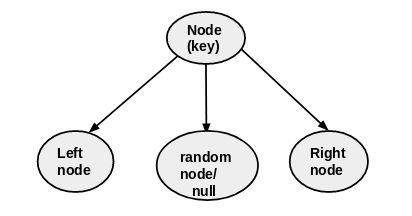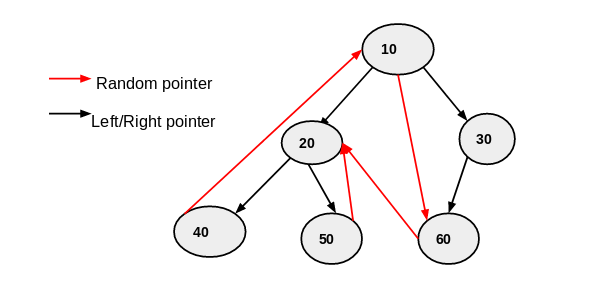×

Search anything:

# Copy a binary tree where each node has a random pointer

#### Problems on Binary Tree Algorithms Data Structures HashingGet this book -> Problems on Array: For Interviews and Competitive Programming

Reading time: 30 minutes | Coding time: 10 minutes

In this article, we will explore an algorithm to copy a binary tree with an additional pointer which points to any random node in the tree. This makes it challenging as a simple traversal will fail as the random pointer may point to a node which we have not yet encountered and hence, we will not be able to copy it.

• Each node of the binary tree has following structure .
1. key(value)
2. left pointer
3. right pointer
4. random pointer
• random pointer point to any random node of binary tree or it can point to null .
•Example of a random pointer Binary tree:We will explore two techniques:

• Naive solution using 2 traversals
• Efficient solution using hashing

## Naive solution: 2 traversals

The naive solution is to traverse the tree two times.

The first time we will traverse it using a standard traversal technique like inorder or preorder and use only the left and right pointers. This way we will copy the entire tree with every random pointers being null.

The idea of the second traversal is to fill up the random pointers. In the second traversal, we will go to each node using standard traversal techniques and copy the random pointer. This will not give any error as all nodes have been copied initially.

At this point, we have copied the entire tree with 2 traversals.

## Efficient solution: Hashing

• To copy a binary tree with random pointer effective solution is hashing.
• We can copy binary tree by maintain a hash table for each node of given binary tree.

## Steps to copy binary tree using random pointer

1. Create a map to store mappings from a node to its clone
2. Recursively traverse the binary tree. store key,left and right pointer of each node into hash table.
• copynode.key=treenode.key
• copynode.left=treenode.left
• copynode.right=treenode.right
• map[treenode]=clonenode
3. update random pointers from the original binary tree into the map
• copynode.random=map[treenode].random

## Implementation

``````import java.util.HashMap;
import java.util.Map;
class Node
{
int data;
Node left,right,random;
Node(int data)
{
this.data=data;
}
};
public class randompointer {
public static void main(String[] args) {
// TODO Auto-generated method stub
Node root = new Node(10);
root.left = new Node(20);
root.right = new Node(30);
root.left.left = new Node(40);
root.left.right = new Node(50);
root.right.left = new Node(60);
root.random = root.right.right;
root.left.left.random = root;
root.left.right.random = root.right.left.random;
root.right.left.random = root.left;
root.random = root.left.right;
System.out.println("original tree:");
preorder(root);
Node copy = copytree(root);
System.out.println("copied tree:");
preorder(copy);
}
public static Node copyleftrightpointer(Node treenode,Map<Node,Node>m)
{
if (treenode == null)
return null;
Node copynode=new Node(treenode.data);
m.put(treenode, copynode);
copynode.left=copyleftrightpointer(treenode.left,m);
copynode.right=copyleftrightpointer(treenode.right,m);
return copynode;
}
public static void copyrandompointer(Node treenode,Node copynode,Map<Node,Node>m)
{
if (copynode==null)
return;
copynode.random=m.get(treenode.random);
copyrandompointer(treenode.left,copynode.left,m);
copyrandompointer(treenode.right,copynode.right,m);
}
public static Node  copytree(Node root)
{
if (root == null)
return null;
Map<Node,Node> m=new HashMap();
Node newtree =copyleftrightpointer(root,m);
copyrandompointer(root,newtree,m);
return newtree;
}
public static void preorder(Node root)
{
if (root == null) {
return;
}
preorder(root.left);
System.out.print(root.data + " -> (");
System.out.print((root.left != null ? root.left.data : "X") + ", ");
System.out.print((root.right != null ? root.right.data : "X") + ", ");
System.out.println((root.random != null ? root.random.data : "X") + ")");
preorder(root.right);
}
}
``````

## Code Explanation:

Original tree• first create map to store mappings from node to its clone(call copytree mathod).
• next we create an new instance(new tree) of node class (which is binary tree) in which we store the left,right,random pointer and data of each node of original tree.
• to copy leftright pointer call copyleftrightpointer() method .
• In this method we put the original node as key and copy node as value in map.
• copy the left and right pointer from original node. to do that we recursively call this method .
• at the end fo this method we get copied tree with data and left-right pointer.
• When all leftrightpointer were copied came back to coptree method. then call copyrandompointer() method.
• to copy random pointer call copyrandompointer() method.
• from the hash table we get the rndom pointer .
• we recursively call copyrandompointer() method to copy random pointer of left-right and node.
• when all random pointers were copied came back to copytree method and return newtree(copied tree).

## Time Complexity :

Time complexity of above implementation is O(n), with linear space O(N)#### Akshay Gopani

Intern at OpenGenus (2019) | Bachelor of Technology (2017 to 2021) in Information and Communication Technology at Ahmedabad University